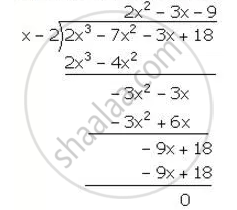Share

# Factorise the Expression F (X) = 2x3 – 7x2 – 3x + 18. Hence, Find All Possible Values of X for Which F(X) = 0. - Mathematics

Course

#### Question

Factorise the expression f (x) = 2x3 – 7x2 – 3x + 18. Hence, find all possible values of x for which f(x) = 0.

#### Solution

f(x)=2x^3-7x^2-3x+18

for x =2,

f(x)=f(2)=2(2)^3-7(2)^2-3(2)+18

=16-28-6+18=0

"Hence, (x-2) is a factor of f(x) "∴ 2x^3-7x^2-3x+18=(x-2)(2x^2-3x-9)

=(x-2)(2x^2-6x+3x-9)

=(x-2)[2x(x-3)+3(x-3)]

=(x-2,(x-3)(2x+3)

now, f(x)=0

⇒2x^3-7x^2-3x+18=0

⇒(x-2)(x-3)(2x+3)=0

⇒x=2, 3, (-3)/2

Is there an error in this question or solution?